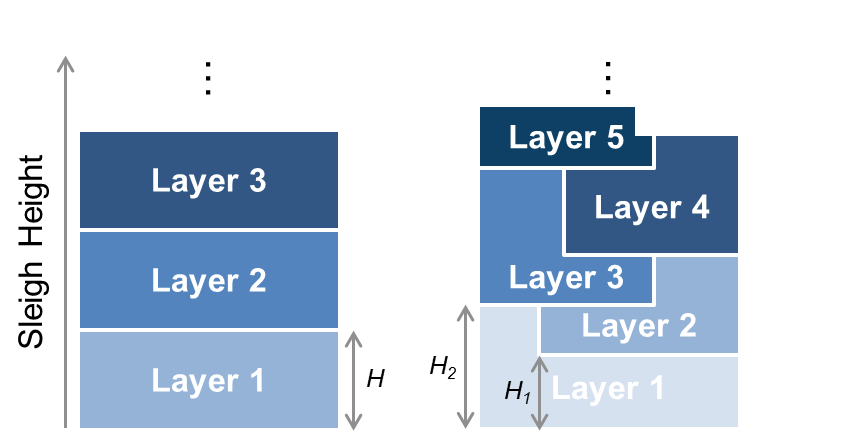# Packing Santa’s Sleigh

Kristen's pick this week is Packing Santa's Sleigh by Alfonso Nieto-Castanon. Hi, my name is Kristen, and this is my first post for the Pick of the Week as a guest blogger. My background is in statistics, machine learning, and data science, which are the areas I support as an Education Technical Evangelist at MathWorks. I use MATLAB every day to support faculty and students at universities around the world as they use MATLAB to teach and conduct research. The most exciting part of my job is seeing the diverse problems that are solved with MATLAB! Today, I’m going to talk about one example... Over the holidays, MathWorks sponsored a Packing Santa's Sleigh competition on Kaggle, a platform for predictive modeling and data analytics competitions. Congratulations to Alfonso, who had the top MATLAB submission and scored second place overall! (Alfonso is no stranger to winning MATLAB contests – see his MATLAB Programming Contest wins here and number one rank on Cody here.)

### Contents

#### Problem Statement

Participants received the $x$-, $y$-, and $z$-dimensions of an ordered list of one million presents. To solve the problem, they were required to pack the presents onto a 1000 x 1000 sleigh, minimizing the total height of the sleigh and keeping the presents as close to the original order as possible. Presents could be rotated as long as they remained parallel to the $x$-, $y$-, and $z$-axes. (You might recognize this as a holiday version of the three-dimensional bin packing problem.)

#### Evaluation Criteria

The solutions were judged on the compactness of packing (minimized height) and present order (delta from original order). The actual evaluation metric $M$ was calculated by $M = 2 \cdot max_{i}(z_{i}) + \sigma(\Gamma)$, where $z_{i}$ = $z$-coordinate of the $i$th present, $\sigma(\Gamma) = \sum_{i=1}^{N_{p}} |i-\Gamma_{i}|$, $\Gamma$ = order the presents appear, and $\Gamma_{i}$ = the Present ID in the $i$th position. The ideal present order is $\Gamma_{ideal} = 1,2,3,\dots,N_{p}$, so that $\sigma(\Gamma_{ideal}) = 0$, where $N_p$ is the total number of presents.

#### Winning MATLAB Solution

A naïve solution might be to simply pack the presents in reverse order from the bottom to the top. Each layer would have a height $H$, the next layer would start at that height, and the process would be repeated until all of the presents are in the sleigh. This would optimize the present order, but leaves much room to reduce the height of the sleigh. Alfonso's solution implements a similar layer-based approach, but he adds a creative twist to how individual layers are defined: He divided the presents in each layer into two sets based on their heights, $H_{1}$ (Set 1) and $H_{2}$ (Set 2). His algorithm keeps the original order of the presents by packing from the bottom up and reverses the solution at the end. Figure 1 shows a naïve layering approach (on the left) and a generalization of Alfonso's solution (on the right).After the first layer, each layer starts partially occupied by presents from the layer below it. The process for each layer looks like this:
1. Select random $H_{1}$ and $H_{2}$ values, where $H_{2}$ was constrained to be greater than or equal to $H_{1}$, and $H_{1}$ was constrained to be greater than or equal to $H_{2}$ - $H_{1}$ from the previous layer.
2. Partition the presents into Set 1 and Set 2. Presents with all sides greater than H1 are put in Set 2. Of the remaining presents, ones that would fit at or below the maximum height are separated into the two sets based on the set for which they minimize the wasted volume $^1$.
3. Estimate the expected volume density $^2$, assuming a given surface density $^3$, based on the partitions of the presents. If the expected volume density is above the current best solution, implement a 2-d solver to place the presents in the layer. If successfully converged, adaptively increase the surface density value; otherwise, decrease the value.
4. This process is repeated until there are no longer any combinations of $H_{1}$ and $H_{2}$ that result in a higher volume density.
After all of the layers are packed, the algorithm compacts them further by attempting to lower each present vertically, if there is room. Finally, the sleigh is flipped upside down to improve the optimal volume density and achieve the optimal order. This got Alfonso his winning score. $^{1,2,3}$ Alfonso provides the definitions of these terms, as well as a more detailed description with additional tricks and nice visualization of his solution, on the competition forum. You can see other Kaggle competitions that he's won here.

Congratulations to Alfonso, the other competition winners, and all 362 competing teams!